How does helix bias work?

Recommended PostsMax Help only says that at bias 1 the turns are forced to one side, at -1 to the other side and at 0 all the turns are evenly distributed. I need to know on what formula or calculation the bias is based.

Basically I’m building a 1 turn helix radius1=radius2=15 height=123. Move it to world origin. Switch viewport to front. The center of this helix changes as the bias changes. :?Yeah, but how? Based on what relation?

If you set bias to 0.5 the center of our turn is at x=-15 y=0 z=120.28208 which is extremely close to the top. How is this calculated?

I want the center of my turn set at z=92.25 which is at 75% of the entire helix height (half the distance between its center and top). According to this, bias should be set at 0.111111111. How is this determined?

Is it a function of radius1 and 2, or of height? :unsure:My guess is that we are dealing with a ratio with an exponential factor based on its parameters. I want this formula. How is the bias affected if the two radii are different?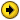My input values are segment AB and segment AC along with basic helix parameters. From these I want to determine/calculate the bias value.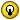Expressed in other terms, how do I get from 0.75 to 0.11111 ?

Can anybody tell what is under the hood of helix bias?

Autodesk 3dsMax 2013 64-bit version 15 product update 6

Acer aspire V3-571G-53214G50Makk, Ivy Bridge Core i5-3210M @ 2.5GHz(turbo boost 3.1GHz), Nvidia GeForce GT 630M (2GB VRAM), 8 GB RAM DDR3 1333MHz, Win 7 Ultimate 64-bit

Share on other sitesOk. I finally got it! This is what I did:

1. Insert the 3ds Max 2013 installation DVD.

2. Select Install Tools & Utilities

3. Select Autodesk 3ds Max 2013 SDK and install

4. Go to the installation directory “maxsdk/samples/objects” and open the file helix.cpp

5. The helix bias is calculated from line 439 to line 445

6. The for loop at line 435 repositions all the helix points and their coordinates according to the bias. I’m only interested in the z position at line 445 > float z = height * hpct. But I want only one z position at a specific x-axis value where it is at minimum which is -15 in my case. This is calculated at line 447 > float x = r * (float)cos(angle).

7.This means that the term cos(angle) in line 447 must be -1 so x = -r. This only happens when angle is -180 or –pi.

8. „angle” is defined at line 446 > float angle = totalRadians * pct

9. Now what is the variable totalRadians?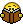IT'S DEFINED IN ANOTHER PLACE AT LINE 626 > totalRadians = TWOPI *turns * ((direction == DIR_CCW) ? 1.0f : -1.0f); which basically means that totalRadians= +/-2*pi*turns. It’s negative when the helix is CW.

10. The term "pct" in line 446 of the angle variable is calculated at line 436 > float pct = (float)i / fPoints; The “i” variable is the current for loop index value iterated for each helix point at line 435. The fPoints variable is calculated at line 433 > float fPoints = (float)points , where points variable is calculated above at line 430> int points = (int)(turns * (float)POINTS_PER_TURN); where POINTS_PER_TURN is a global variable set at 40 in line 23 which determines the smooth of the helix curve. So I have to force this equality:

totalRadians*pct = -pi +/-2*pi*turns*(i/(turns*40))=-pi. In short this happens at i=20 so pct= i/(t*40)=1/(2*t). Which is half the way in the for loop of the helix.

11. Then at lines 440 > hpct = 1.0f -r(float)pow(1.0f - pct, power ); and at line 443 > hpct = (float)pow(pct,power); we seem to calculate a variable hpct which is a factor in our z.position. NOW WHERE IS “POWER” DEFINED?,IT IS DEFINED AT LINE 631!> power = bias * 9.0f + 1.0f; and at line 634 > power = -bias * 9.0f+ 1.0f;

12. FINALY THE FORMULA IS: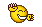Z.POS=helix.height*(1-(1-1/(2*helix.turns))^(9*helix.bias-1)) when bias>0Z.POS=helix.height*(1/2*helix.turns)^(1-9*helix.bias) when bias

Since I know the z.pos and I WANT THE BIAS I have to remember that if x=b^y then y=log(base: b, number: x) so from the above we calculate and go straight for the result:BIAS=(log(base: (1-1/2t) ; number: (h-p/h))-1)/9 when p>h/2BIAS=(1-log(base: 1/2t ; number: p/h))/9 when p

And t – helix turns ; p – z. position of the center point we know; h – helix height

Autodesk 3dsMax 2013 64-bit version 15 product update 6

Acer aspire V3-571G-53214G50Makk, Ivy Bridge Core i5-3210M @ 2.5GHz(turbo boost 3.1GHz), Nvidia GeForce GT 630M (2GB VRAM), 8 GB RAM DDR3 1333MHz, Win 7 Ultimate 64-bit

Share on other sitesActually the above formulas work well only for turns=1. When turns=0.5 they fail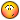. However since in my case I'm only interested in the HELIX CENTER POSITION the "turns" parameter is no longer required.

Thus, the relations are like following:

p = h*(1-(0.5)^(9b+1)) when b>0

p = h*(0.5)^(1-9b) when b

and in reverse:

b=(log( base:0.5 ; nr: ((h-p)/h))-1)/9 when p>h/2

b=(1-log( base:0.4 ; nr: p/h))/9 when p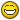where:

p is z.position (the height of the helix center point)

h is the helix height

b is the helix bias

Share on other sites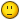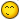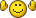Join the conversation

You can post now and register later. If you have an account, sign in now to post with your account.
Note: Your post will require moderator approval before it will be visible.Reply to this topic...

×   Pasted as rich text.   Paste as plain text instead

Only 75 emoji are allowed.